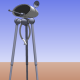## Ultrasonic Toggle Switch Source Code (Arduino)

```
// ultrasonic toggle switch
// Joe Schembrie aka 'Engineer Zero'

// the circuit for this sketch consists of a yellow and green LED and an ultrasonic distance sensor (pinger).
// when a hand approaches the pinger, the yellow light comes on with the approach.
// when the hand gets close enough, the value of green is toggled.
// IE, if the green LED was off, it turns on.  If it was on, it turns off.

// to see this in action, visit https://engineerzero.wordpress.com/2014/06/01/ultrasonic-toggle-switch/

// The green LED toggles only once while the hand is near.
// IMPORTANT
// The hand must be retracted far enough so that the yellow light goes off
// for the green LED to be toggled again.  This prevents 'bouncing.'

// NOTE:  In some respects, this device seems to work best when the yellow light is disconnected.
// NOTE ON NOTE:  I wrote the above note a couple years ago AND DON'T REMEMBER WHY.

// Now for inclusion of the library.
// the NewPing.h library is available on the Internet.
// I did not write it.  I cannot answer questions about it.
// It comes with documentation on the Internet.

#include <NewPing.h>

#define TRIGGER_PIN  11  // Arduino pin tied to trigger pin on the ultrasonic sensor.
#define ECHO_PIN     10  // Arduino pin tied to echo pin on the ultrasonic sensor.
#define MAX_DISTANCE 200 // Maximum distance we want to ping for (in centimeters). Maximum sensor distance is rated at 400-500cm.
NewPing sonar(TRIGGER_PIN, ECHO_PIN, MAX_DISTANCE);

// now to stuff that I wrote.
// here are some very important variables

// dis is distance of hand to sensor.  greenstate is state of green light.
// toggle is a variable that gives approval to toggle.
// Note that 'toggle' does not by itself trigger a toggle.
// It only gives 'approval to toggle.'

unsigned int dis;
int greenstate = 0;
int toggle = 10;

void setup() {
// put your setup code here, to run once:

pinMode(2,OUTPUT);  // yellow is 2
pinMode(3,OUTPUT);  // green is 3
pinMode(11,OUTPUT); // pins 10 and 11 are used by NewPing
pinMode(10,INPUT);

digitalWrite(2,LOW);
digitalWrite(3,LOW);

}

void loop() {
// put your main code here, to run repeatedly:

// NewPing stuff . . .

delay(50);

unsigned int uS;
uS = sonar.ping();

dis = (uS/US_ROUNDTRIP_CM);

// . . . end of NewPing stuff.  Now onto my stuff:

// the following code sets the zone value according to distance.
//
// after toggling, toggle approval is de-activated but
// is re-activated if the hand is retracted to zone 0.
// this prevents nuisance toggling (bouncing).

int zone;

// zone = 0 means the hand is far enough away that the sensor doesn't react to it.
// toggle approval is granted.
// I set the distance at 40 cm.  You can experiment with distances as you please.

if(dis>=40){
zone = 0;
toggle=10;
}

// zone = 1 means the hand is close enough to turn on yellow light.

if(dis<40){
zone = 1;
}

// zone = 2 means the hand is close enough to toggle the green light.
// the 10 cm distance is open to your choice.

if (dis<10){
zone = 2;
}

// Now, I vaguely remember that the reason for the following test had something
// to do with the quirkiness of the sensor.  Sometimes it fails to read and
// reports a zero distance.  The following test prevents that from causing problems.

if(dis<2){
zone = 0;
toggle = 10;
}

// now we take care of yellow light.
// It's pretty simple.  If you're within a certain distance, it's on.  Otherwise, off.
//

if(zone==0){
digitalWrite(2,LOW);
}

if(zone>0){
digitalWrite(2,HIGH);
}

// we toggle the variable greenstate (and, most importantly, the green light)
// if we're in zone 2 and toggle is 10.
//
// why these values?  It will become apparent in later context.
//
// zone 2 means we're close enough to toggle.
//
// toggle = 10 means go ahead and toggle.
// toggle = 0 means don't toggle.
//
// greenstate = 0 means the green light is OFF.
// greenstate = 100 means the green light is ON.
//
// 'toggle' is a variable that 'approves' toggling the green light
// only ONCE when you're close to the sensor.  It's then set to 0
// and your hand has to move away in order for it to reset to 10.
// this prevents bouncing.

int k = 0;

// the k variable is a handy way to avoid making a complex IF statement.
// Maybe it's me, but sometimes I just can't get multi-condition IF statements
// to work on the Arduino.
//
// So instead, I use individual IF statements to create flag variables
// and then add the flag variables together to create a 'master flag variable'
// which I can then test in a simple one-variable conditional statement.
//
// So k is for 'kludge.'  But it works . . . .

k = toggle + zone + greenstate;

// k = 112 means that the toggle (=10) is approved, we're in toggle zone (=2) ,
// and the greenstate (=100) indicates the green light is ON.

if(k==112){
greenstate = 0;
digitalWrite(3,LOW);
toggle = 0;
}

// k = 12 means that the toggle (=10) is approved, we're in toggle zone (=2) ,
// and the greenstate (=0) indicates the green light is OFF.

if(k==12){
greenstate = 100;
digitalWrite(3,HIGH);
toggle = 0;
}

// these two statements then toggle the green light
// (and circuits activated by the same pin).
//
// note that for all other values of k, no action is taken.

// And that's all there is to it!!!

}

```1.Edward Comer says: